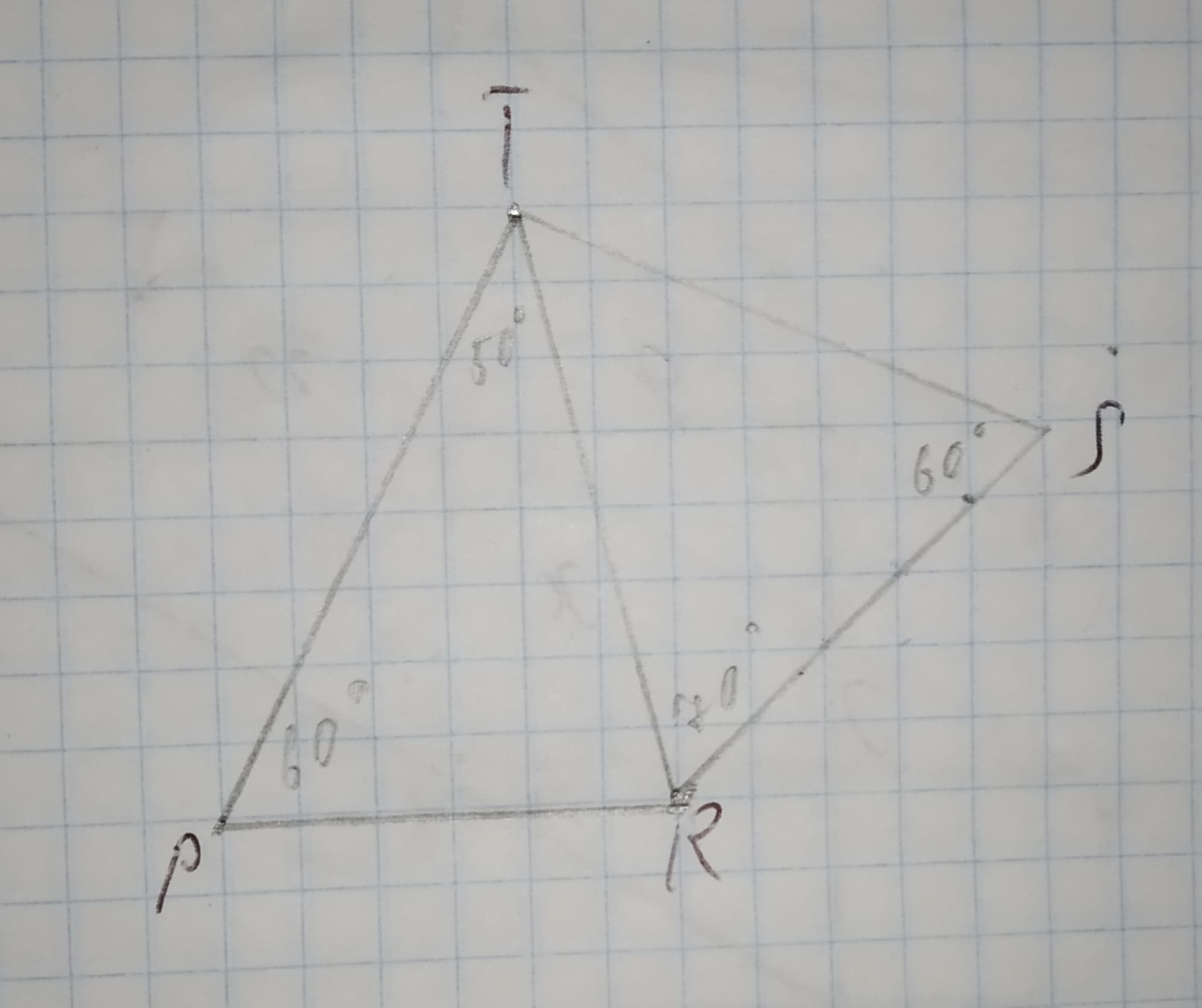# Decide whether the two triangles must be congruent. If so, write the congruence and name the postulate used. If not. write no congruence can be deduced.12210203482.jpgnagasenaz 2020-11-05 Answered

Decide whether the two triangles must be congruent. If so, write the congruence and name the postulate used. If not. write no congruence can be deduced.You can still ask an expert for help

## Want to know more about Congruence?

• Questions are typically answered in as fast as 30 minutes

Solve your problem for the price of one coffee

• Math expert for every subject
• Pay only if we can solve itmhalmantus

Given figure is:Sum of angles in a triangle is ${180}^{\circ }$.
$In\mathrm{△}TPR$
${50}^{\circ }+{60}^{\circ }+\mathrm{\angle }TRP={180}^{\circ }$
$\mathrm{\angle }TRP={70}^{\circ }$
Step 4
$In\mathrm{△}TSR$,
${60}^{\circ }+{70}^{\circ }+\mathrm{\angle }TRP={180}^{\circ }$
$\mathrm{\angle }TRP={50}^{\circ }$
$In\mathrm{△}TRP\phantom{\rule{1em}{0ex}}\text{and}\phantom{\rule{1em}{0ex}}\mathrm{△}TSR$,
$\mathrm{\angle }TPR=\mathrm{\angle }TSR\left(Each{60}^{\circ }\right)$
$\mathrm{\angle }PTR=\mathrm{\angle }STR\left(Each{50}^{\circ }\right)$
TR=TR (Common)
Therefore, $\mathrm{△}TPR\stackrel{\sim }{=}\mathrm{△}TSR$ (BY AAS-congruence rule)/
Hence, both triangles are congruent.